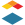## Is it possible to randomize in a quiz questions that are worth different points?Community Participant

Suppose you have 10 questions, 5 of them worth two points, 3 of them worth four points, and the other 2 worth 10 points.

Is there a way to create a quiz with these 10 questions where the order of questions (altogether) is fully randomized (shuffled)?

I know questions in a question group are randomized. However, all questions within a group are worth a fixed number of points. In this example, I could use 3 question groups. However, the order of groups is fixed within a quiz. ☹️  Therefore, randomization is not for the 10 questions "in the same bucket".

To me this is a very basic thing that we should be able to do: randomize all questions in a quiz + have questions worth different number of points.  Is there a workaround or tip/hack to do that? 🤞

Labels (1)
• ### Canvas

1 SolutionInstructure

Unfortunately, with Classic quizzes that is not an option. The questions would need to be inside a group to be randomized.

However, that has been addressed in our new quizzes.

On new quizzes, we have an option called "shuffle questions" that will randomize all the questions of the quiz.

Here is a guide on how to create a quiz using the New quizzes tool.

Here is a guide about the settings you can set up on that quiz.